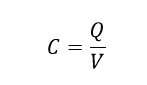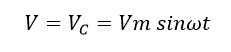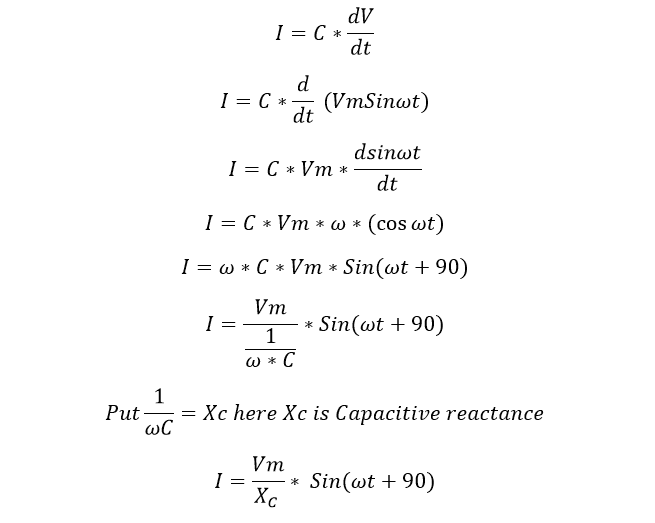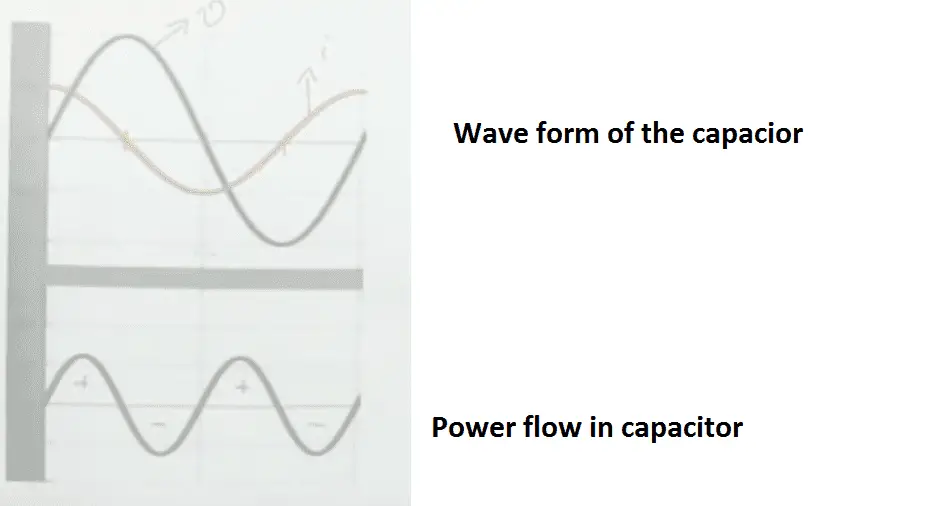# Why Capacitor Has Leading Power Factor

### Why Capacitor Has Leading Power Factor:

• Both the wave should be sinusoidal
• Both signal should be same frequency
• Both signal must be written in positive amplitude

First, consider two charged parallel plates Capacitance is nothing but a ratio of Charge in a one plate to potential difference between two plates is called capacitance. Capacitance is a property measure of capacitor. C is the capacitance of the two plates, Q is charge and V is potential difference, hence Capacitance C is equal toConsider an electric circuit which has Vc is the voltage across the capacitor, I is the sinusoidal current flow through the capacitor and V is the applied voltage here applied voltage equal to capacitor voltage which meansCurrent flow through theNow we get current and Voltage magnitude let draw the phasor diagram. Here you can see the current leads ahead the voltage by 90deg. due to the leading nature of current, we get leading power factor in capacitor.

Learn More:   Why India has 50 Hz Power System and US has 60 Hz 110 Volts Power System

Lets see the power flow in capacitor:A pure capacitor loads the power stored is equal to the power return back to the source. Hence A pure capacitor does not store any power.